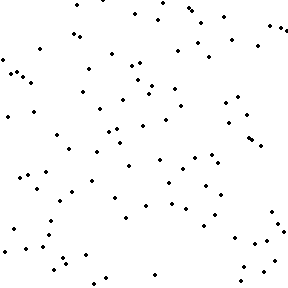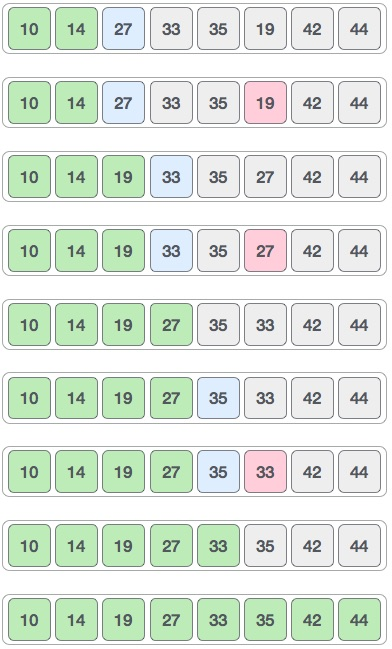# Selection Sort Basic Information

suggest change

Selection sort is a sorting algorithm, specifically an in-place comparison sort. It has O(n2) time complexity, making it inefficient on large lists, and generally performs worse than the similar insertion sort. Selection sort is noted for its simplicity, and it has performance advantages over more complicated algorithms in certain situations, particularly where auxiliary memory is limited.

The algorithm divides the input list into two parts: the sublist of items already sorted, which is built up from left to right at the front (left) of the list, and the sublist of items remaining to be sorted that occupy the rest of the list. Initially, the sorted sublist is empty and the unsorted sublist is the entire input list. The algorithm proceeds by finding the smallest (or largest, depending on sorting order) element in the unsorted sublist, exchanging (swapping) it with the leftmost unsorted element (putting it in sorted order), and moving the sublist boundaries one element to the right.

Pseudo code for Selection sort:

``````function select(list[1..n], k)
for i from 1 to k
minIndex = i
minValue = list[i]
for j from i+1 to n
if list[j] < minValue
minIndex = j
minValue = list[j]
swap list[i] and list[minIndex]
return list[k]``````

Visualization of selection sort:Example of Selection sort:Auxiliary Space: `O(n)`

Time Complexity: `O(n^2)`